The Tactile Sensing with Light Propagation in a Four-Layer Slab Waveguide for Breast Tumor DetectionReview Article

Austin J Biomed Eng. 2021; 6(1): 1043.

# The Tactile Sensing with Light Propagation in a Four-Layer Slab Waveguide for Breast Tumor Detection

Lee J-H*

University of Keimyung, School of Medicine, South Korea

*Corresponding author: Jong-Ha Lee, University of Keimyung, School of Medicine, South Korea

Received: April 06, 2021; Accepted: May 19, 2021; Published: May 26, 2021

## Abstract

Light propagation in a 4 layer slab waveguide is considered. The supported modes are of higher orders than zero, with a larger node concentration toward the layers with larger refractive index. Additionally, we consider the geometric optics approximation to describe very thick waveguides. Light can be coupled from LED by direct focusing with a lens, and coupling can be optimized by introducing a transverse displacement in order to focus it on the layers with larger refractive index. Further optimization can be done by tilting the lens and bringing in some degree of coma aberrations.

Keywords: Refractive index; Geometric optics; Optical waveguide

## Introduction

Optical waveguide is the fundamental element that interconnects various devices of an optical integrated circuit, just as a metallic strip does it in an electrical integrated circuit. Light travels in optical waveguides in form of the guided modes, which are the only form, allowed propagating in waveguides according to electromagnetic laws [1,2]. The modes represent stable distribution of electromagnetic field inside the waveguide. Each mode has a different field profile depending on the geometry and properties of the waveguide [3,4].

Normally used in optics are the dielectric waveguides, which are made of a number of optical layers usually a few wavelengths thick and having different refractive indexes . Having the refractive index larger than that of the surrounding medium allows for light to be trapped inside the waveguide due to total internal reflection. The layers can be made of glass, liquid crystals, organic polymer, and could even have an externally controlled refractive index .

Light is directed into the waveguide using an optical coupler. A number of solutions are used for that purpose, such as direct coupling, prism coupling, grating coupling, and tapered coupling. The simplest possible is direct focusing with a lens, in which light is focused onto the waveguide edge with an optical lens . In order to couple light into the waveguide one needs to excite its modes, which usually means only exciting the basic one. Coupling efficiency will be determined by how well the input wave’s profile matches that of the mode. If the profiles are matched and there is no discontinuity, light from the source will just continue propagating into the waveguide. However, if there is a discontinuity, only some portion of light would be coupled, while the rest would be diffracted away [8,9].

In this paper we consider light propagation in a four-layer planar dielectric slab waveguide. The problem is formulated analytically and develops into a complete set of equations in Section 3. A specific case of a waveguide is considered as a demonstration of general properties of the structure in Section 4. The geometric optics approximation describing very thick waveguides is given in Section 5. Optical coupling and optimization is considered in Section 6. Section 7 concludes the results of the paper.

## The Waveguide

As shown in Figure 1, the 4-layer waveguide is made of glass, on top of which three Polydimethylsiloxane (PDMS) have been formed. The waveguide is illuminated through its edges by LED light sources.

Layer 1: PDMS, refractive index 1 and height h1

Layer 2: PDMS, refractive index 2 and height 2

Layer 3: PDMS, refractive index 3 and height 3

Layer 4: Borosilicate glass plate, refractive index 4 and height 4

The layers are positioned in the order of increasing refractive index, so 1 > n­2 > n­3 > n­4. Since the waveguide is surrounded by air, having a lower refractive index than any of the layers of the waveguide, the incident light directed into the waveguide remains contained within it .

Light propagates in the direction z, and the layers are positioned in the direction x. The layer boundaries have coordinates .

x = 0,

x = a1 = h1,

x = a2 = h1 + h2,

x = a3 = h1 + h2 + h3,

x = a4 = h1 + h2 + h3 + h4.

Size of the waveguide is 3 cm, which is much larger than the typical layer thickness. We therefore are going to assume an infinite dimension in planar direction y.

## Analytic Formulation

Light propagation in a dielectric waveguide is described by the wave equation.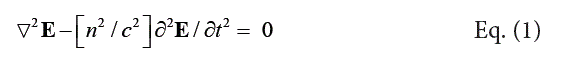Here E(x, y, z, t) is the electric field, n is the refractive index, and c is the speed of light in vacuum. A similar equation is valid for the magnetic field H(x, y, z, t):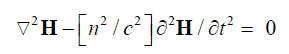For monochromatic waves with frequency ω the solution of Eq. (1) has the form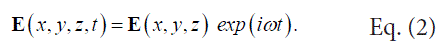Given that form, the spatial distribution of electric field E(x, y, z) follows this equation.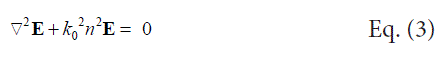where k0 is the wave vector in vacuum: k0 = ω / c. Since the waveguide is uniform in z-direction, we can look only for planar wave solutions: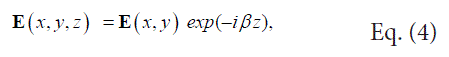where β is the propagation constant. This is analogous to free wave propagation in a form of a plane wave in bulk medium . The propagation constant β has the dimensions and the physical meaning of wave vector k in a medium with effective refractive index neff:

k=k0neff,

Considering that the problem is independent of direction y, the field varies only across dimension x.

E(x, y) = E(x).

Further, let us first consider the solution for the transverse y-component of the electric field, representing the TE mode. For that purpose, let us assume:

E(x) = e(x) j,

where j is the unit vector along the direction y. By substituting that into Eq. (3) we can reduce it to the following ordinary differential equation:

d2e/dx2 + [k02n2 − β2] e = 0                 Eq. (5)

This equation has to hold throughout all regions including the slabs and the air

Region 0:              x < 0                       d2e/dx2 + [k02n02 − β2] e = 0

Region 1:              0 < x < a1              d2e/dx2 + [k02n12 − β2] e = 0

Region 2:              a1< x < a2              d2e/dx2 + [k02n22 − β2] e = 0

Region 3:              a2< x < a3              d2e/dx2 + [k02n32 − β2] e = 0

Region 4:              a3< x < a4              d2e/dx2 + [k02n42 − β2] e = 0

Region 5:              x > a4                     d2e/dx2 + [k02n02 − β2] e = 0               Eq. (6)

The refractive index n0 is the refractive index of the medium surrounding the waveguide, in this case air n0=1, and the remaining indexes follow the inequality 1 > 2 > 3 > 4 > n0.